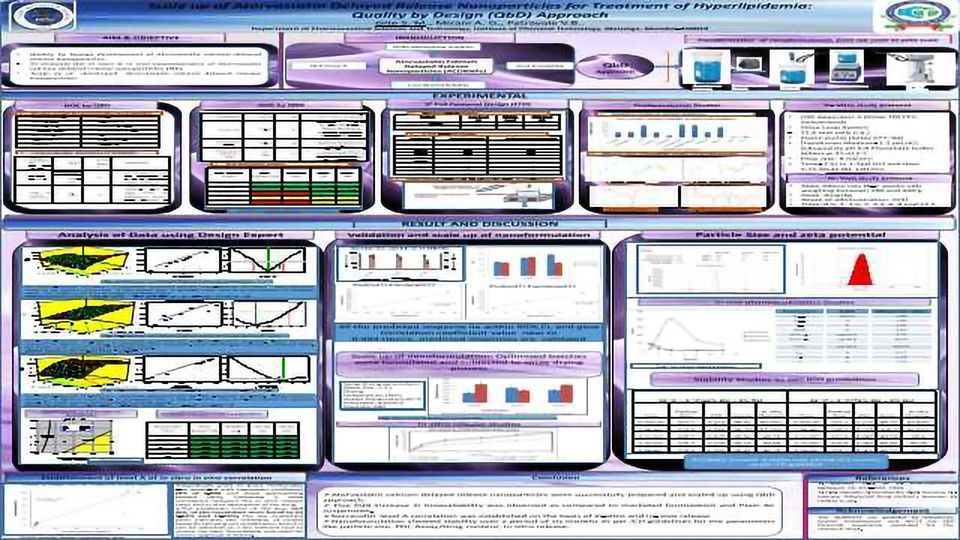We use cookies to provide you with a better experience. You can read our Cookie Policy here.

# Scale up of Atorvastatin Delayed Release Nanoparticles for Treatment of Hyperlipidemia: Quality by Design (QbD) ApproachIntroduction: Atorvastatin calcium (AC) is a selective, competitive inhibitor of HMG-CoA reductase. Atorvastatin is BCS class II molecule and rapidly degrades in acid environment and thereby results in poor bioavailability. To overcome this problem, delayed release nanoparticle of atorvastatin were developed using industrial scalable QbD approach.
Experimental Methods:
Preparation of Atorvastatin calcium delayed release nanoparticles (ACDRNPs): Nanoparticles were developed using rapid solvent diffusion technology that can be easily transferred for industrial scale.
Quality Target Product Profile (QTPP) and Critical Quality Attributes (CQA) of ACDRNPs: As per ICH guidelines QTPP and CQAs were established on the basis of preliminary experiments.
Risk Assessment Analysis: The risk assessment studies were carried out to identify the Critical formulation parameters (CFP)/ Critical process parameters (CPP) for ACDRNPs.
32 Full Factorial Design (FFD):
A 32 full factorial design was used in this study where 2 factors evaluated each at 3 levels; experimental trial was performed at all 9 possible combinations. Drug: Polymer ratio (X1) and Stabilizer Concentration (X2) were selected as independent variables based on risk assessment analysis.
Optimization using desirability function:
Desirability function was calculated for particle size and encapsulation efficiency, based on the acceptance limit for response variable.
Validation of optimized formulations:
The three checkpoint formulations were evaluated to validate the design
Result and Discussion:
Response surface analysis is the graphical mapping of effect of independent variables on dependent variables. The response surface analysis was carried out using 2D plot (contour plot) and 3D plot to explain the effect of independent variables on dependent variable.
Mathematical modeling:
Mathematical relationships generated using multiple regression analysis for the studied response variables are expressed in equation (1), (2).
Particle size (Y1):
P Size (Y1) = +659.65 +345.0 * X1-37.29* X2……… (1)
The equation (1) showed that the coefficient X1 bears a positive sign, indicating that the Y1 increases with increase in X1 concentration. X2 bears a negative sign, indicating that the Y1 decreases with increase in concentration of Stabilizer (X2).
EE(Y2)= +62.12 -58.05 * X1-14.16* X2 -4.43* X1 * X2-90.50* X12 +6.40* X22…………(2)
The result of equation (2) shows that the negative sign of X1 indicates that increased concentration of X1 shows increase in Y2, negative sign of X2 indicates that decreased concentration of X2 shows increase in Y2.